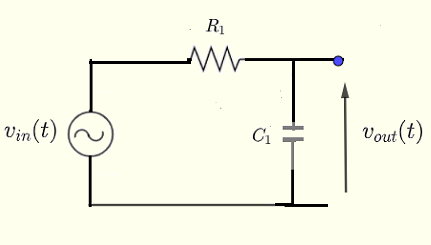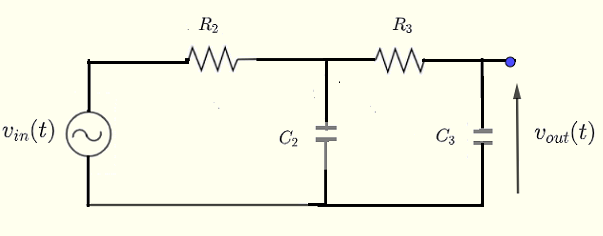# Low Pass Filter Transfer Function Graphing Calculator

    

A calculator and grapher to calculate and graph the magnitude and phase of the the transfer function of low pass filters of the first and second order is presented.
In what follows, $j$ is the imginary unit and $\omega$ is the angular frequency given by $\omega = 2 \; \pi \; f$ where $f$ is the frequency of the input signal and $s = j \; \omega$
This calculator and grapher are related to the transfer function of low pass filters investigated in this site.

## Transfer Function of First Order Low Pass FilterThe transfer function of a first order low pass filter is given by
$H(s) = \dfrac{ 1}{1 + R_1 \; C_1 \; s }$ or $H(\omega) = \dfrac{ 1}{1 + j \; R_1 \; C_1 \; \omega }$ The magnitude of $H$ is given by $|H(\omega)| = \dfrac{1}{\sqrt{1^2+(R_1 \; C_1 \; \omega)^2}} = \dfrac{1}{\sqrt{1+(R_1 \; C_1 \; \omega)^2}}$ The phase of $H$ is given by $|\Phi(\omega)| = \arctan(0) - \arctan \left(\dfrac{R_1 \; C_1 \; \omega}{1}\right) = - \arctan \left(R_1 \; C_1 \; \omega \right)$ The $- 3 \; \text{dB}$ cutoff frequency defined in transfer function of a first order low pass filter is given by $\omega_c = \dfrac{1}{R_1 C_1}$

## Transfer Function of Second Order Low Pass FilterThe transfer function of a second order low pass filter is given by
$H(s) = \dfrac{1 }{ R_2 R_3 C_2 C_3 \; s^2 + (R_2 C_2 + R_3 C_3 + R_2 C_3) \; s + 1}$ or $H(\omega) = \dfrac{1 }{ 1 - R_2 R_3 C_2 C_3 \; \omega^2 + j \; (R_2 C_2 + R_3 C_3 + R_2 C_3) \; \omega + 1}$ The magnitude and phase are given by $| H(\omega) | = \dfrac{1}{\sqrt{ (1 - A\; \omega^2)^2 + (B\omega)^2 }}$ $\Phi (\omega) = - \arctan \left(\dfrac{ \;B \; \omega }{ 1 - A \omega^2 }\right)$ The $-3 \text{ dB}$ cutoff frequency defined in transfer function of a second order low pass filter is given by $\omega_c = \dfrac{1}{\sqrt A} \sqrt { 1 - 2 r^2 + \sqrt{ 4 r^4 - 4 r^2+ 2 } }$ where $r$ is also defined in transfer function of a second order low pass filter .

## Use of the Calculator and Grapher

This calculator accepts values of the resistance $R_1$ and capacitance $C_1$ of the first order low pass filter and also the resistsnces $R_2$, $R_3$ and capaciatnces $C_2$ and $C_3$ of the second order low pass filter.
The calculator gives the transfer functions in terms of $s$ and $\omega$, the magnitudes, the phases and the cutoff frequencies of both filters.
NOTE that the output in blue is for the first order and the output in red is for the second order.
Enter the resistances and the capacitances then press "calculate".
The graphs of $20 \log_{10}{ | H(\omega |}$ and the phases $\Phi(\omega)$ are displayed and the interval $h$ between points may be adjusted using a slider.

 Low Pass Filter First Order Resistance $R_1$ = 100 mΩ Ω KΩ MΩ Capacitance $C_1$ = 50 pF nF μF mF F --------------------------------------------------------------------------------------- Low Pass Filter Second Order Resistance $R_2$ = 10000 mΩ Ω KΩ MΩ Capacitance $C_2$ = 0.1 pF nF μF mF F Resistance $R_3$ = 40000 mΩ Ω KΩ MΩ Capacitance $C_3$ = 0.01 pF nF μF mF F

## Graphing

NOTE Slide or click left, center and right, to either to decrease (left) or increase (right) the interval $h$ in order to have well scaled graphs.